# Difference between revisions of "User:IssaRice/Computability and logic/Diagonalization lemma"

The diagonalization lemma, also called the Godel-Carnap fixed point theorem, is a fixed point theorem in logic.

## Rogers's fixed point theorem

Let$f$ be a total computable function. Then there exists an index$e$ such that$\varphi_e \simeq \varphi_{f(e)}$.

(simplified)

Define$d(x) = \varphi_x(x)$ (this is actually slightly wrong, but it brings out the analogy better).

Consider the function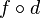$f\circ d$. This is partial recursive, so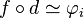$f\circ d \simeq \varphi_i$ for some index$i$.

Now$\varphi_{f(d(i))} \simeq \varphi_{\varphi_i(i)}$ since$f\circ d \simeq \varphi_i$. This is equivalent to$\varphi_{d(i)}$ by definition of$d$. Thus, we may take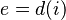$e = d(i)$ to complete the proof.

## Diagonalization lemma

(semantic version)

Let$A$ be a formula with one free variable. Then there exists a sentence$G$ such that$G$ iff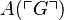$A(\ulcorner G\urcorner)$.

Define$\mathrm{diag}(x)$ to be$\ulcorner C(\ulcorner C\urcorner)\urcorner$ where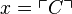$x = \ulcorner C\urcorner$. In other words, given a number$x$, the function$\mathrm{diag}$ finds the formula with that Godel number, then diagonalizes it (i.e. substitutes the Godel number of the formula into the formula itself), then returns the Godel number of the resulting sentence.

Let$B$ be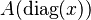$A(\mathrm{diag}(x))$, and let$G$ be$B(\ulcorner B\urcorner)$.

Then$G$ is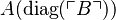$A(\mathrm{diag}(\ulcorner B\urcorner))$, by substituting$x = \ulcorner B\urcorner$ in the definition of$B$.

We also have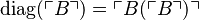$\mathrm{diag}(\ulcorner B\urcorner) = \ulcorner B(\ulcorner B\urcorner)\urcorner$ by definition of$\mathrm{diag}$. By definition of$G$, this is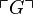$\ulcorner G\urcorner$, so we have$\mathrm{diag}(\ulcorner B\urcorner) = \ulcorner G\urcorner$.

To complete the proof, apply$A$ to both sides of the final equality to obtain$A(\mathrm{diag}(\ulcorner B\urcorner))$ iff$A(\ulcorner G\urcorner)$; this simplifies to$G$ iff$A(\ulcorner G\urcorner)$.

## Comparison table

Step Rogers's fixed point theorem Diagonalization lemma
Theorem statement$\varphi_e \simeq \varphi_{f(e)}$$G \leftrightarrow A(\ulcorner G\urcorner)$
Given mapping$f$$A$
Definition of diagonal function$d(x) = \varphi_x(x)$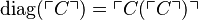$\mathrm{diag}(\ulcorner C\urcorner) = \ulcorner C(\ulcorner C\urcorner)\urcorner$
Composition of given mapping with diagonal function (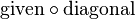$\mathrm{given} \circ \mathrm{diagonal}$)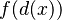$f(d(x))$$A(\mathrm{diag}(x))$
Naming the composition$f\circ d$$B$
Index of composition$i$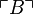$\ulcorner B\urcorner$$d(i)$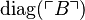$\mathrm{diag}(\ulcorner B\urcorner)$
Expanding using definition of diagonal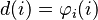$d(i) = \varphi_i(i)$$\mathrm{diag}(\ulcorner B\urcorner) = \ulcorner B(\ulcorner B\urcorner) \urcorner$
Composition applied to own index (i.e. diagonalization of the composition)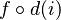$f\circ d(i)$$B(\ulcorner B\urcorner)$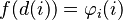$f(d(i)) = \varphi_i(i)$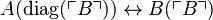$A(\mathrm{diag}(\ulcorner B\urcorner)) \leftrightarrow B(\ulcorner B\urcorner)$
Explicitly showing previous composition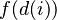$f(d(i))$$A(\mathrm{diag}(\ulcorner B\urcorner))$
Definition of G$\varphi_i(i)$ is$f(d(i))$$G$ is$A(\mathrm{diag}(\ulcorner B\urcorner))$
Renaming index$e = d(i)$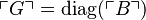$\ulcorner G\urcorner = \mathrm{diag}(\ulcorner B\urcorner)$
Leibniz law to previous row Apply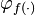$\varphi_{f(\cdot)}$ to obtain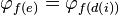$\varphi_{f(e)} = \varphi_{f(d(i))}$ Apply$A(\cdot)$ to obtain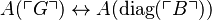$A(\ulcorner G\urcorner) \leftrightarrow A(\mathrm{diag}(\ulcorner B\urcorner))$
Use definition of G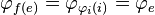$\varphi_{f(e)} = \varphi_{\varphi_i(i)} = \varphi_e$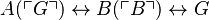$A(\ulcorner G\urcorner) \leftrightarrow B(\ulcorner B\urcorner) \leftrightarrow G$